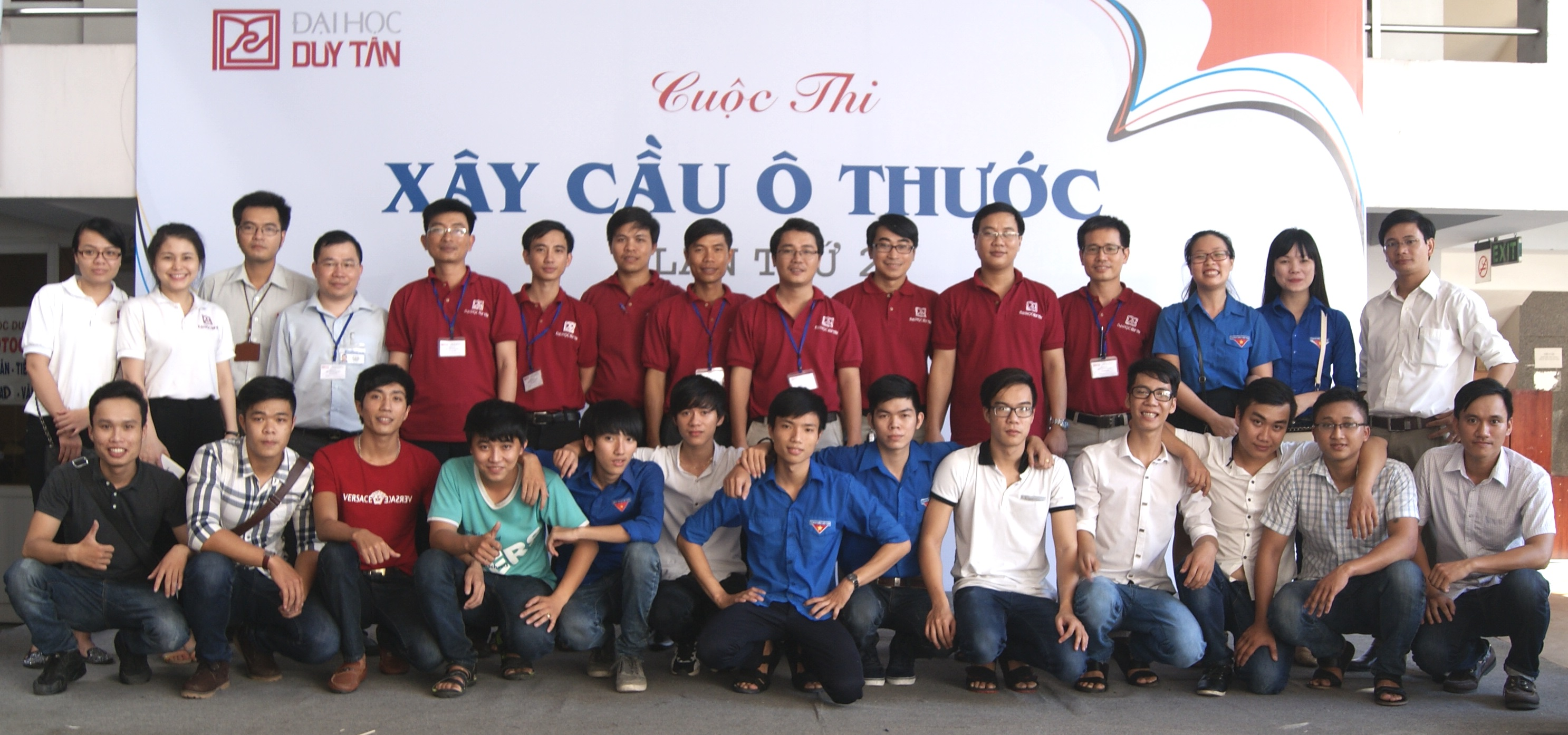Tài liệu học tập

### MEC 316: Bài tập chương 2

• Đăng bởi: nguyenphuocbinh
• Sinh viên
• 18/04/2019

2.1

Consider the soil profile shown in Figure

1. Calculate the variations of s, u, and s’ at points A, B, and C.
2. How high should the groundwater table rise so that the effective stress at C is 111 kN/m2?

2.2

For a sandy soil with Gs=2.68, calculate the critical hydraulic gradient that will cause boiling or quick condition for e = 0.38, 0.48, 0.6, 0.7, and 0.8. Plot the variation of icr with the void ratio.

2.3

Following data are given for a direct shear test conducted on dry sand:

• Specimen dimensions: 63 mm x 63 mm x 25 mm (height)
• Normal stress: 105 kN/m2
• Shear force at failure: 300 N
1. Determine the angle of friction, j
2. For a normal stress of 180 kN/m2, what shear force is required to cause failure?

2.4

Consider the specimen in Problem 2.3.

1. What are the principal stresses at failure?
2. What is the inclination of the major principal plane with the horizontal?

2.5

For a dry sand specimen in a direct shear test box, the following are given:

• Size of specimen: 63.5 mm x 63.5 mm x 31.75 mm (height)
• Angle of friction: 33°
• Normal stress: 193 kN/m2

Determine the shear force required to cause failure

2.6

A strip load of q=43 kN/m2 is applied over a width, B=11 m. Determine the increase in vertical stress at point A located z= 4.6 m below the surface. Given: x = 8.2 m.

2.7

For the embankment loading shown in Figure, determine the vertical stress increases at points A, B, and C.

2.8

Refer to Figure. A flexible rectangular area is subjected to a uniformly distributed load of q=225 kN/m2. Determine the increase in vertical stress at a depth of z =3 m under points A, B, and C.

2.9

Refer to the flexible loaded rectangular area shown in Figure. Determine the vertical stress increase below the center of the loaded area at depths z = 2, 4, 6, 8, and 10 m.

Tải file đính kèm:Phát bằng tốt nghiệpPhan Minh TiếnHoạt động ngoại khóa